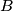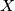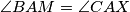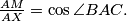### IMO Shortlist 1985 problem 21

Kvaliteta:
Avg: 0,0
Težina:
Avg: 0,0
The tangents at$B$ and$C$ to the circumcircle of the acute-angled triangle$ABC$ meet at$X$. Let$M$ be the midpoint of$BC$. Prove that

(a)$\angle BAM = \angle CAX$, and

(b)$\frac{AM}{AX} = \cos\angle BAC.$
Izvor: Međunarodna matematička olimpijada, shortlist 1985